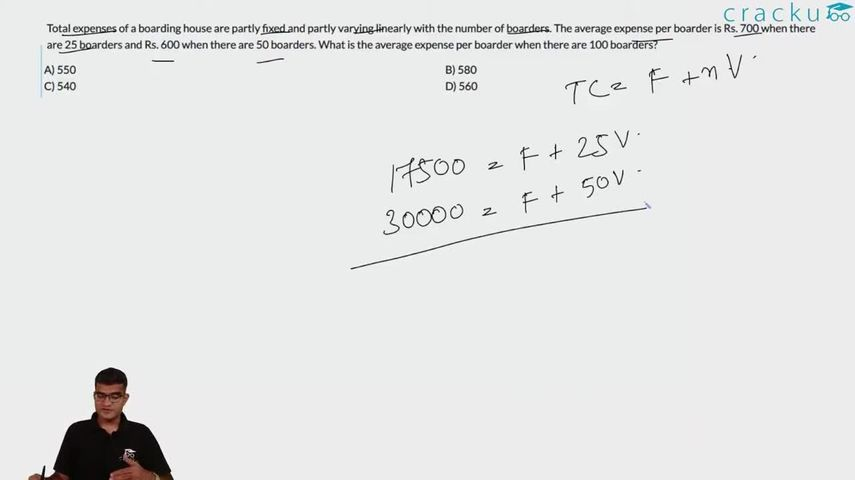Question 7

# Total expenses of a boarding house are partly fixed and partly varying linearly with the number of boarders. The average expense per boarder is Rs. 700 when there are 25 boarders and Rs. 600 when there are 50 boarders. What is the average expense per boarder when there are 100 boarders?

Solution

Let the fixed income be x and the number of boarders be y.

x + 25y = 17500

x + 50y = 30000

=> y = 500 and x = 5000

x + 100y = 5000 + 50000 = 55000

Average expense = $$\frac{55000}{100}$$ = Rs.550.

### View Video Solution